Rational numbers - Definition

Chapter 1 Class 9 Number Systems
Concept wise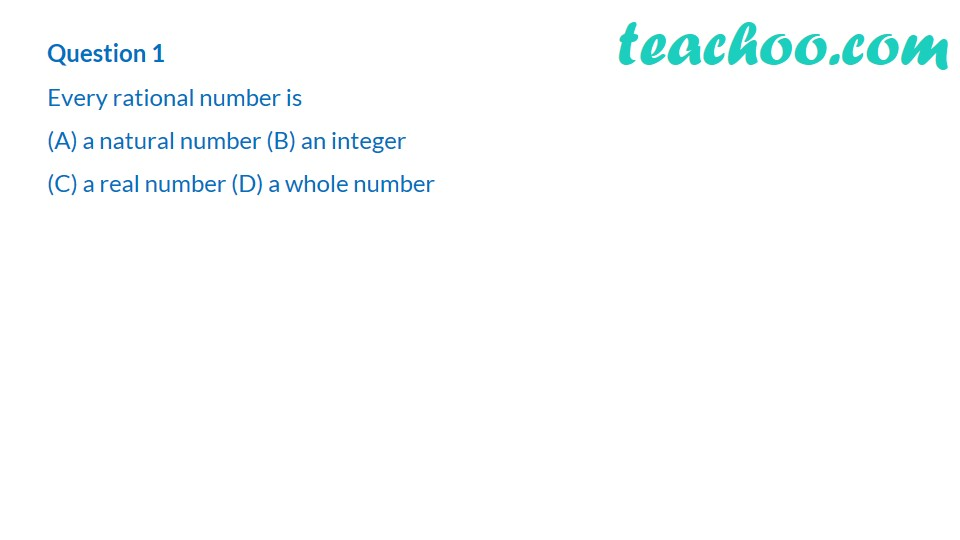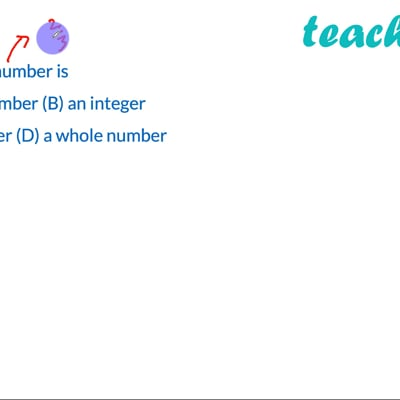This video is only available for Teachoo black users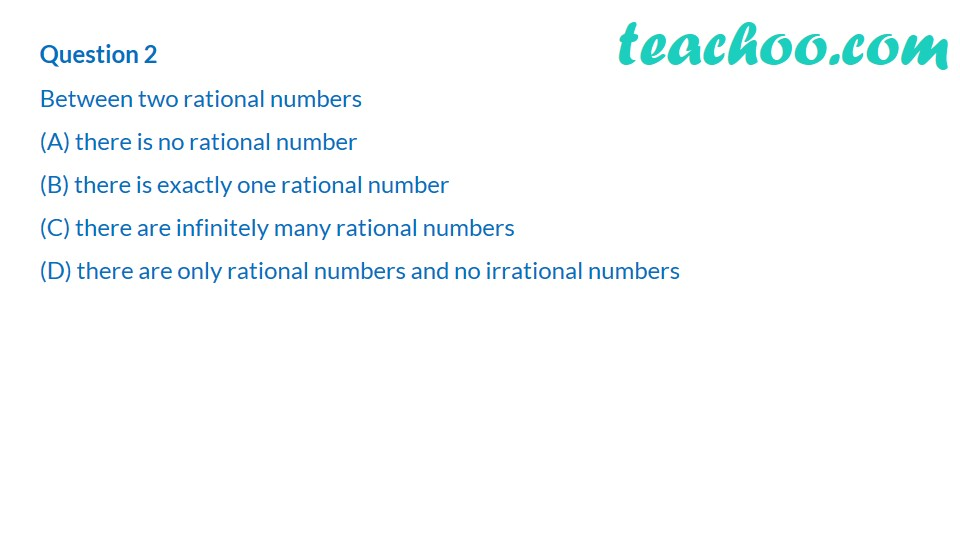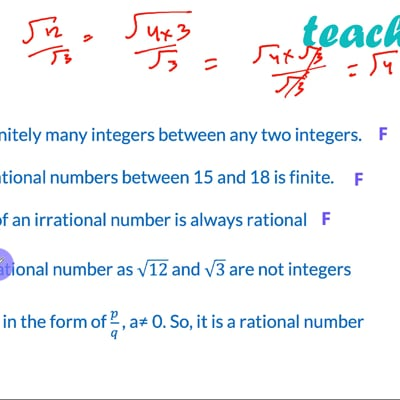This video is only available for Teachoo black users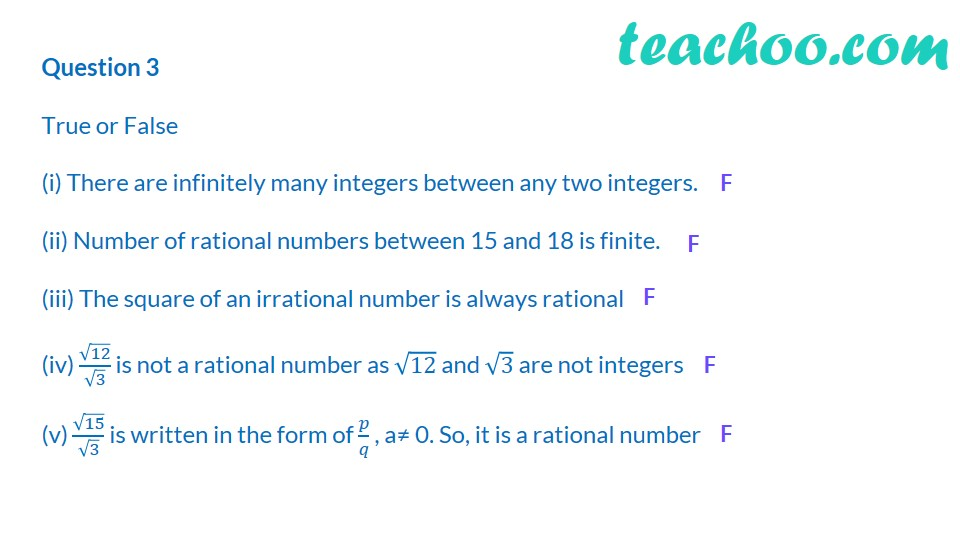This video is only available for Teachoo black users

Maths Crash Course - Live lectures + all videos + Real time Doubt solving!

### Transcript

Question 1 Every rational number is (a) a natural number (b) an integer (c) a real number (d) a whole number Question 2 Between two rational numbers (a) there is no rational number (b) there is exactly one rational number (c) there are infinitely many rational numbers (d) there are only rational numbers and no irrational numbers Question 3 True or False (i) There are infinitely many integers between any two integers. F (ii) number of rational numbers between 15 and 18 is finite. F (iii) The square of an irrational number is always rational F (iv) root12/root3 is not a rational number as root 12 and root 3 are not integers F (v) root 15 / root 3 is written in the form of p/q, a = 0. So, it is a rational number F